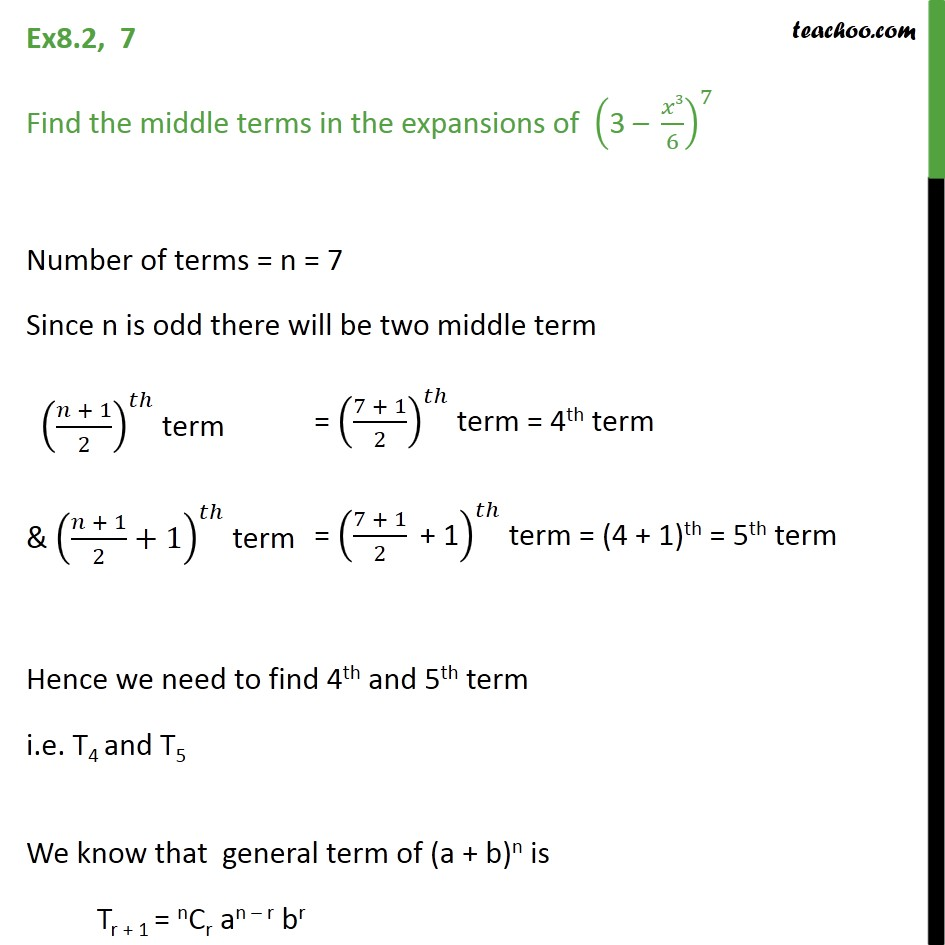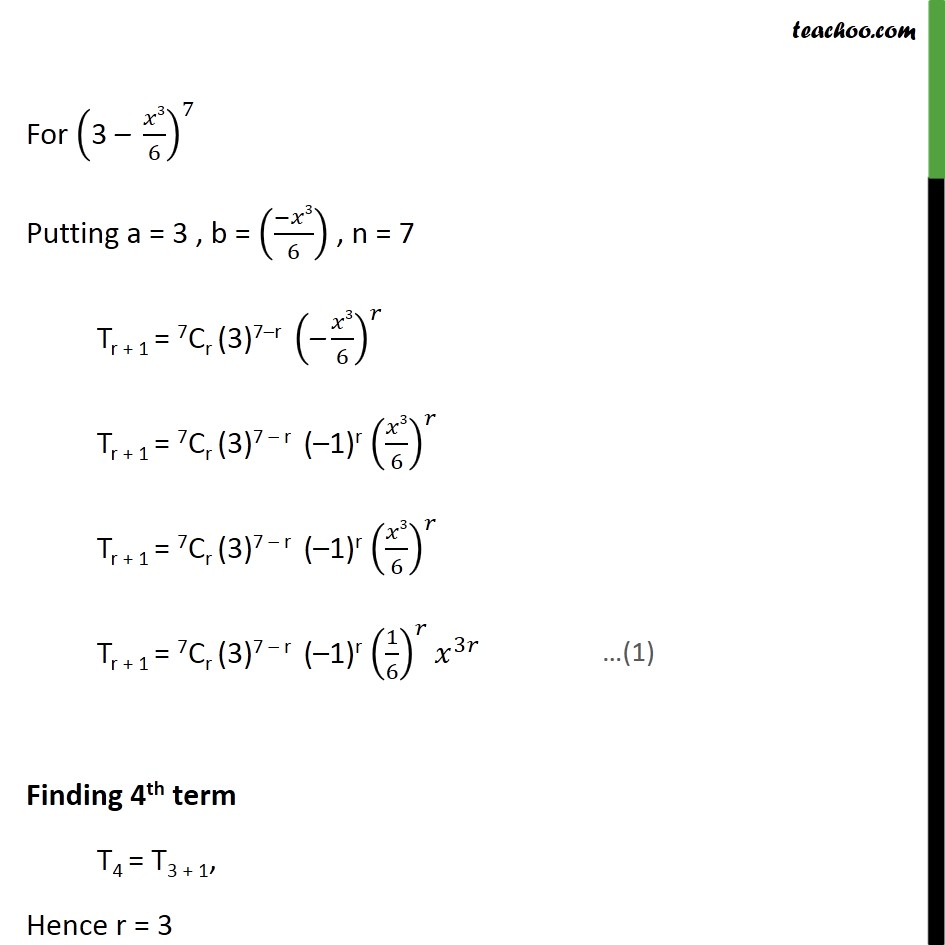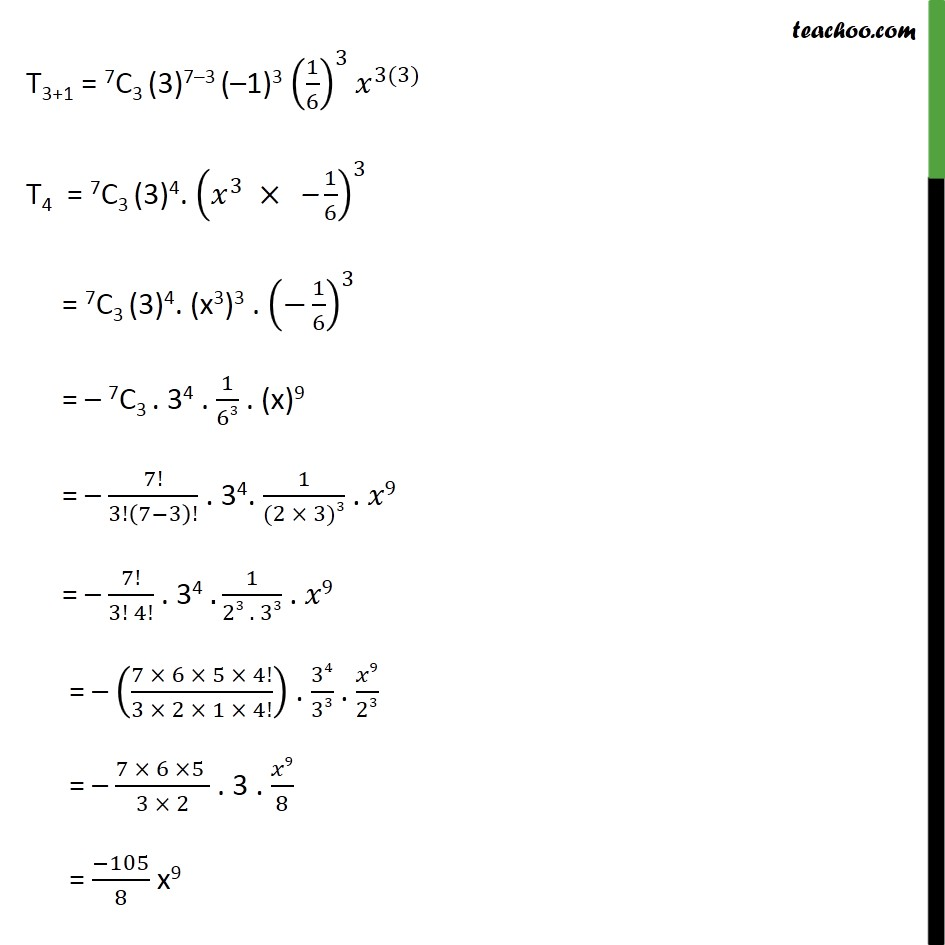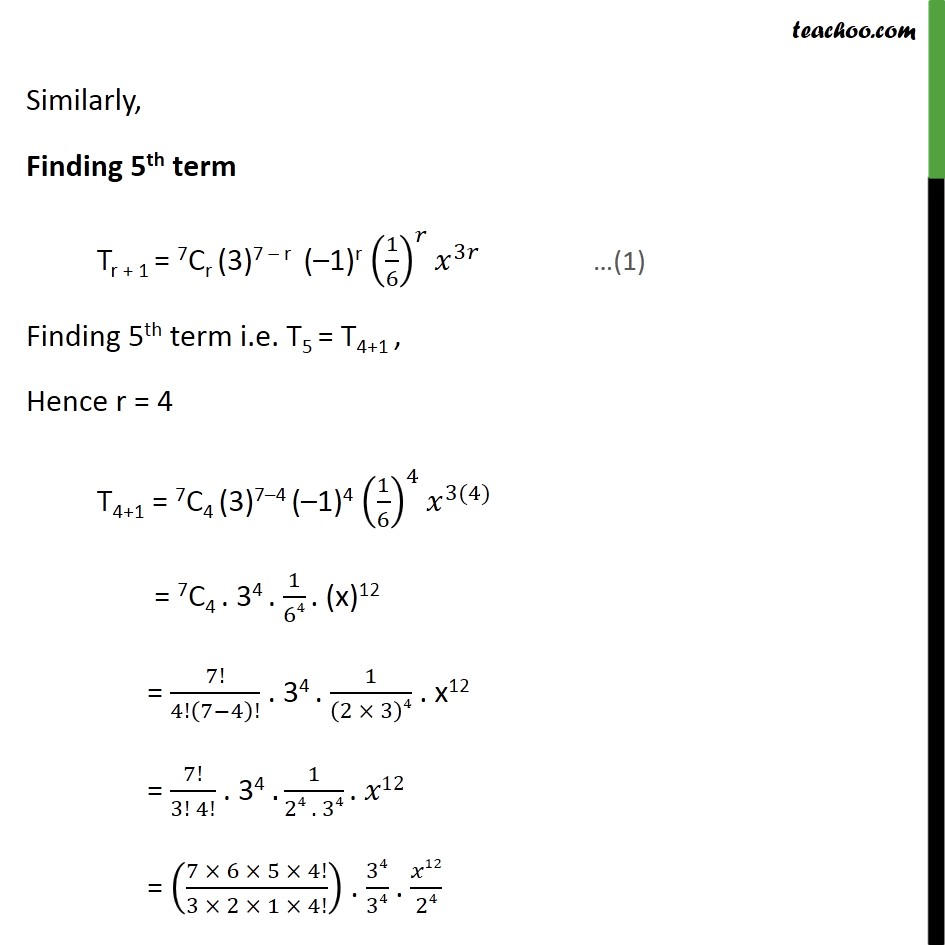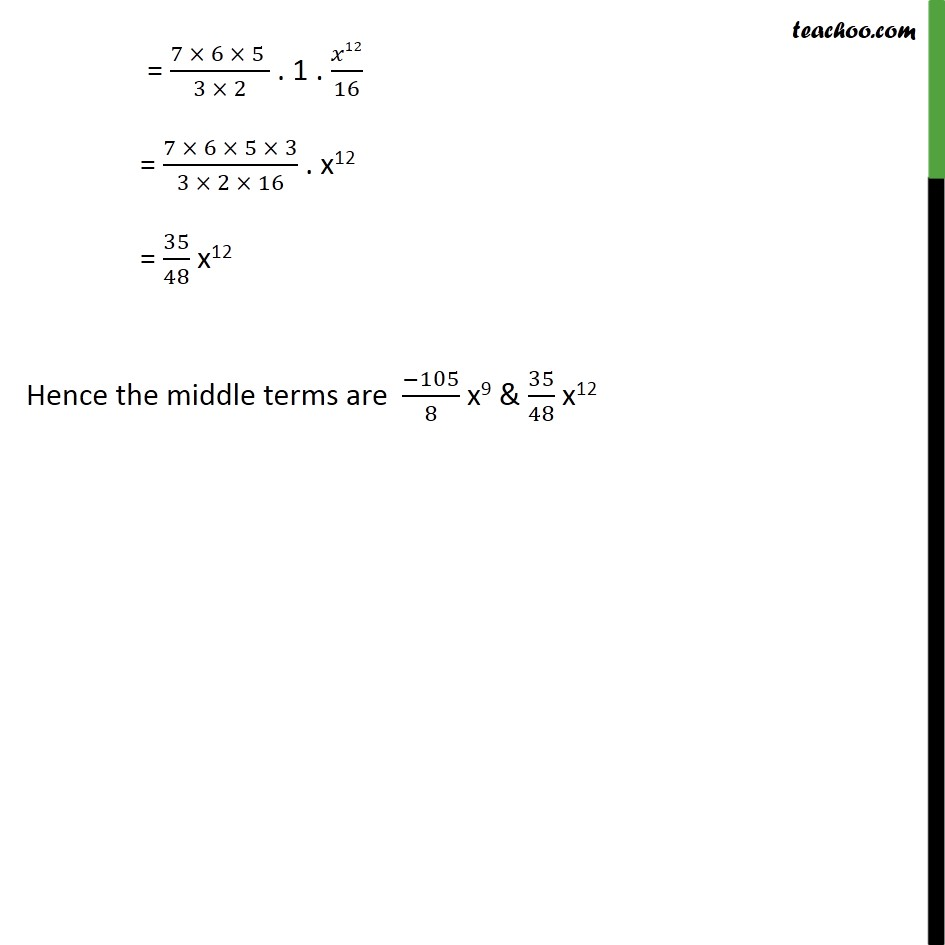1. Chapter 8 Class 11 Binomial Theorem
2. Concept wise
3. Middle term

Transcript

Ex 8.2,7 Find the middle terms in the expansions of ﷐﷐3 – ﷐𝑥3﷮6﷯﷯﷮7﷯ Number of terms = n = 7 Since n is odd there will be two middle term ﷐﷐﷐𝑛 + 1﷮2﷯﷯﷮𝑡ℎ﷯ term & ﷐﷐﷐𝑛 + 1﷮2﷯+1﷯﷮𝑡ℎ﷯ term Hence we need to find 4th and 5th term i.e. T4 and T5 We know that general term of (a + b)n is Tr + 1 = nCr an – r br For ﷐﷐3 – ﷐𝑥3﷮6﷯﷯﷮7﷯ Putting a = 3 , b = ﷐﷐−𝑥3﷮6﷯﷯ , n = 7 Tr + 1 = 7Cr (3)7–r ﷐﷐–﷐𝑥3﷮6﷯﷯﷮𝑟﷯ Tr + 1 = 7Cr (3)7 – r (–1)r ﷐﷐﷐𝑥3﷮6﷯﷯﷮𝑟﷯ Tr + 1 = 7Cr (3)7 – r (–1)r ﷐﷐﷐𝑥3﷮6﷯﷯﷮𝑟﷯ Tr + 1 = 7Cr (3)7 – r (–1)r ﷐﷐﷐1﷮6﷯﷯﷮𝑟﷯﷐𝑥﷮3𝑟﷯ Finding 4th term T4 = T3 + 1, Hence r = 3 T3+1 = 7C3 (3)7–3 (–1)3 ﷐﷐﷐1﷮6﷯﷯﷮3﷯﷐𝑥﷮3(3)﷯ T4 = 7C3 (3)4. ﷐﷐﷐𝑥﷮3﷯ × –﷐1﷮6﷯﷯﷮3﷯ = 7C3 (3)4. (x3)3 . ﷐﷐−﷐1﷮6﷯﷯﷮3﷯ = – 7C3 . 34 . ﷐1﷮63﷯ . (x)9 = – ﷐7!﷮3!﷐7−3﷯!﷯ . 34. ﷐1﷮(2 × 3)3﷯ . 𝑥9 = – ﷐7!﷮3! 4!﷯ . 34 .﷐1﷮23 . 33﷯ . 𝑥9 = – ﷐﷐7 × 6 × 5 × 4!﷮3 × 2 × 1 × 4!﷯﷯ . ﷐34﷮33﷯ . ﷐𝑥9﷮23﷯ = – ﷐7 × 6 ×5 ﷮3 × 2﷯ . 3 . ﷐𝑥9﷮8﷯ = ﷐−105﷮8﷯ x9 Similarly, Finding 5th term Tr + 1 = 7Cr (3)7 – r (–1)r ﷐﷐﷐1﷮6﷯﷯﷮𝑟﷯﷐𝑥﷮3𝑟﷯ …(1) Finding 5th term i.e. T5 = T4+1 , Hence r = 4 T4+1 = 7C4 (3)7–4 (–1)4 ﷐﷐﷐1﷮6﷯﷯﷮4﷯﷐𝑥﷮3(4)﷯ = 7C4 . 34 . ﷐1﷮64﷯ . (x)12 = ﷐7!﷮4!﷐7−4﷯!﷯ . 34 . ﷐1﷮﷐2 × 3﷯4﷯ . x12 = ﷐7!﷮3! 4!﷯ . 34 .﷐1﷮24 . 34﷯ . 𝑥12 = ﷐﷐7 × 6 × 5 × 4!﷮3 × 2 × 1 × 4!﷯﷯ . ﷐34﷮34﷯ . ﷐𝑥12﷮24﷯ = ﷐7 × 6 × 5 ﷮3 × 2﷯ . 1 . ﷐𝑥12﷮16﷯ = ﷐7 × 6 × 5 × 3﷮3 × 2 × 16﷯ . x12 = ﷐35﷮48﷯ x12 Hence the middle terms are ﷐−105﷮8﷯ x9 & ﷐35﷮48﷯ x12

Middle term